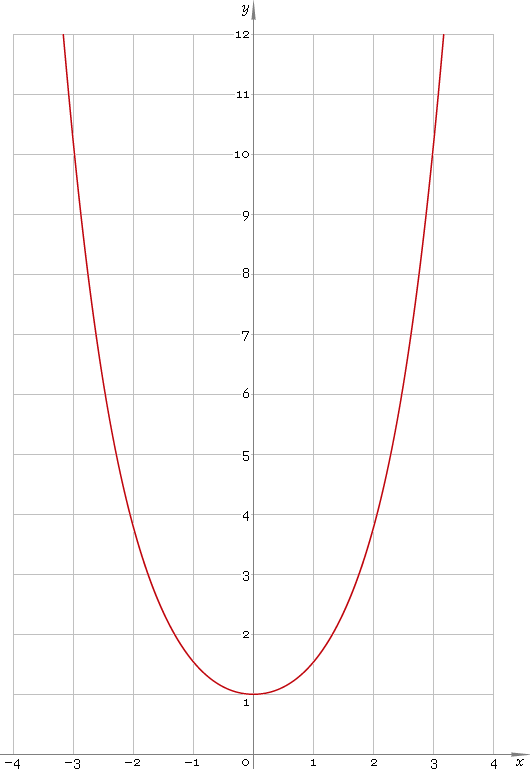The Art of Mathematics

# cosh or ch — hyperbolic cosine function

## 1. Definition

Hyperbolic cosine is defined as

coshx ≡ (ex + ex) /2

## 2. Graph

Hyperbolic cosine is symmetric function defined everywhere on real axis. Its catenary graph is depicted below — fig. 1.Fig. 1. Graph of the hyperbolic cosine function y = coshx.

Function codomain is range [1, +∞).

## 3. Identities

Base:

cosh2x − sinh2x = 1

Relation to exponential function:

sinhx + coshx = ex
coshx − sinhx = ex

By definition:

coshx ≡ 1 /sechx

Property of symmetry:

cosh−x = coshx

Half-argument:

cosh(x/2) = √[(coshx + 1) /2]
coshx = [1 + tanh2(x/2)] /[1 − tanh2(x/2)]

Doulbe argument:

cosh(2x) = sinh2x + cosh2x
cosh(2x) = 2 cosh2x − 1
cosh(2x) = 2 sinh2x + 1

Triple argument:

cosh(3x) = 4 cosh3x − 3 coshx

cosh(4x) = 8 cosh4x − 8 cosh2x + 4 = 8 cosh2x sinh2x + 1 = 8 sinh4x + 8 sinh2x + 1

Power reduction:

cosh2x = (cosh(2x) + 1) /2
cosh3x = (cosh(3x) + 3 coshx) /4
cosh4x = (cosh(4x) + 4 cosh(2x) + 3) /8
cosh5x = (cosh(5x) + 5 cosh(3x) + 10 coshx) /16

Sum and difference of arguments:

cosh(x + y) = coshx coshy + sinhx sinhy
cosh(xy) = coshx coshy − sinhx sinhy

Product-to-sum:

coshx coshy = [cosh(x + y) + cosh(xy)] /2
sinhx coshy = [sinh(x + y) + sinh(xy)] /2

Sum-to-product:

coshx + coshy = 2 cosh[(x + y) /2] cosh[(xy) /2]
coshx − coshy = 2 sinh[(x + y) /2] sinh[(xy) /2]

## 4. Derivative and indefinite integral

Hyperbolic cosine derivative:

cosh′x = sinhx

Indefinite integral of the hyperbolic cosine:

∫ coshx dx = sinhx + C

where C is an arbitrary constant.

## 5. How to use

To calculate hyperbolic cosine of the number:

``cosh(−1);``

To get hyperbolic cosine of the complex number:

``cosh(−1−i);``

To get hyperbolic cosine of the current result:

``cosh(rslt);``

To get hyperbolic cosine of the number z in calculator memory:

``cosh(mem[z]);``

## 6. Support

Hyperbolic cosine of the real argument is supported in free version of the Librow calculator.

Hyperbolic cosine of the complex argument is supported in professional version of the Librow calculator.